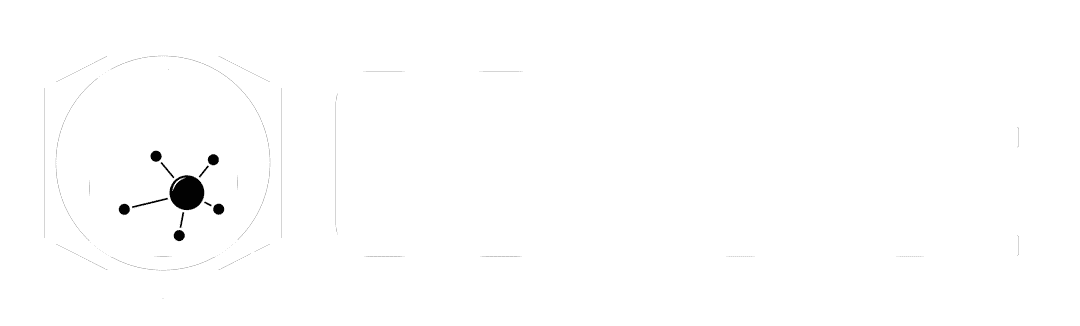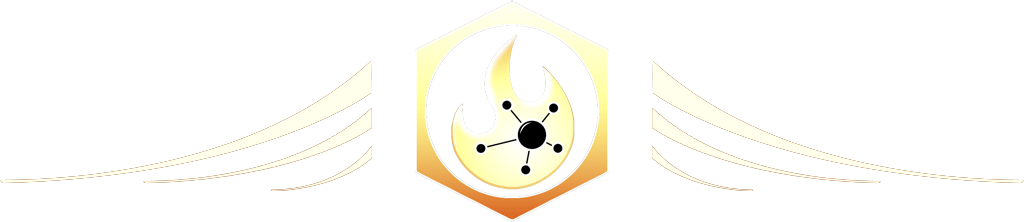# Divide

The Ultimate Houdini node reference

# Visit the Node Bible

### Divide

The Divide SOP allows you to re-topologize a mesh in various ways.
Drag to resize

Summary:

The Divide SOP can change your topology in a variety of ways.  This can happen by triangulating a mesh, smoothing it (by subdividing), and fixing N-gons (polygons with more than 4 sides), concave polygons, or triangulating meshes.

Drag to resize

General Parameters:

Group:
Convex Polygons:
-  First things first - You need to understand what a “convex” polygon is. Look at the image below: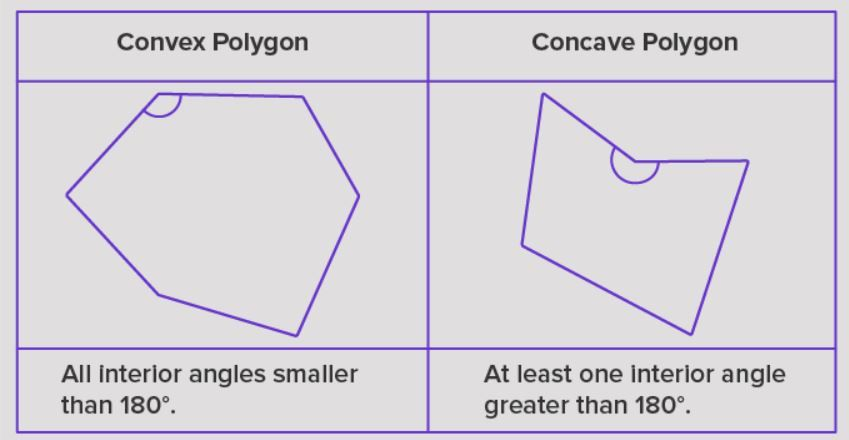-  Great!  Now, when you turn on "Convex Polygons" it will make sure that you no longer have any concave polys by dividing it.  Most of the time, you'll want to leave this parameter on because concave polygons can cause a variety of issues.

-  Also, note that the second input of the Divide SOP is expecting "rest" geometry.  This "rest" geometry is typically a character that is sitting in it's non-moving rest pose.  When the "Convex Polygons" is turned on and you have the rest geo plugged in, it will only do this calculation based on the position of the polygons of the rest mesh.  In addition to that, also make sure that your point IDs are the same between the rest and deformed geo if you go this route.

Maximum Edges:

-  This will divide a polygon if it is over this many edges.  In practice, this will allow you to triangulate meshes.

Triangulate Non-Planar:

-  This parameter will determine whether or not each polygon is "non-planar."  According to the docs, "A non-planar primitive is a primitive whose vertices do not all lie on the same plane."  To better see this, take a look at the image below: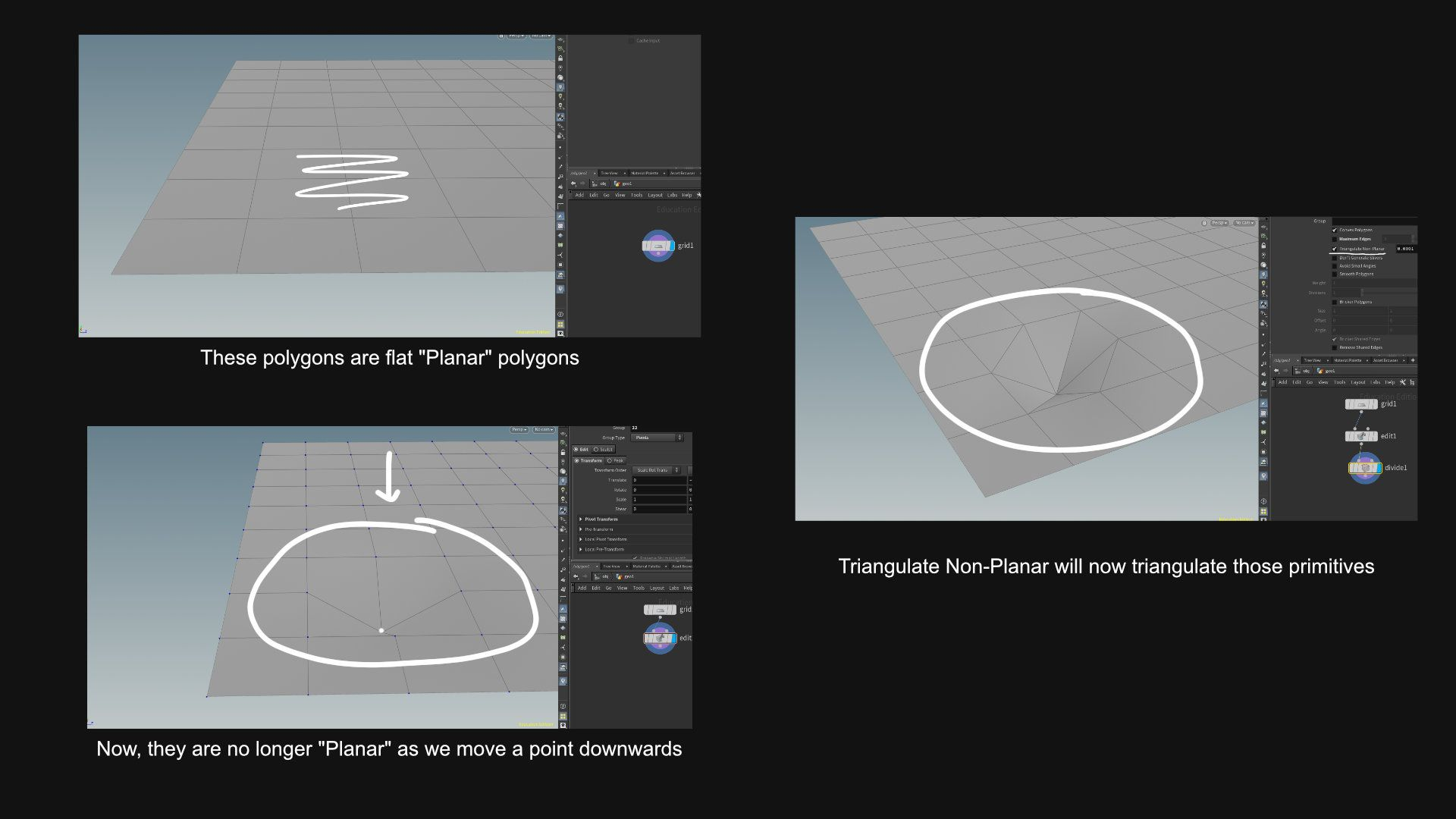Don't Generate Slivers:

-  This will prevent tiny little triangles from being generated if the remesh is triangulating a mesh.  IMPORTANT - this can create bad geometry with open meshes and disconnected polygons which will cause major problems with RBD, vdb operations, booleans, and more.  In practice, it is wise to avoid this parameter and rely more on the "Avoid Small Angles" parameter instead.

Avoid Small Angles:

-  The Divide SOP will attempt to avoid small angles if it is triangulating a mesh.  I'm not sure why this optional, but it will improve the quality of the divide sop, and it's best to leave it on.

Smooth Polygons:

-  This will provide a subdividing algorithm that subdivides and smooths the polygons.  If we are to subdivide the mesh described in the diagram above, we would get this: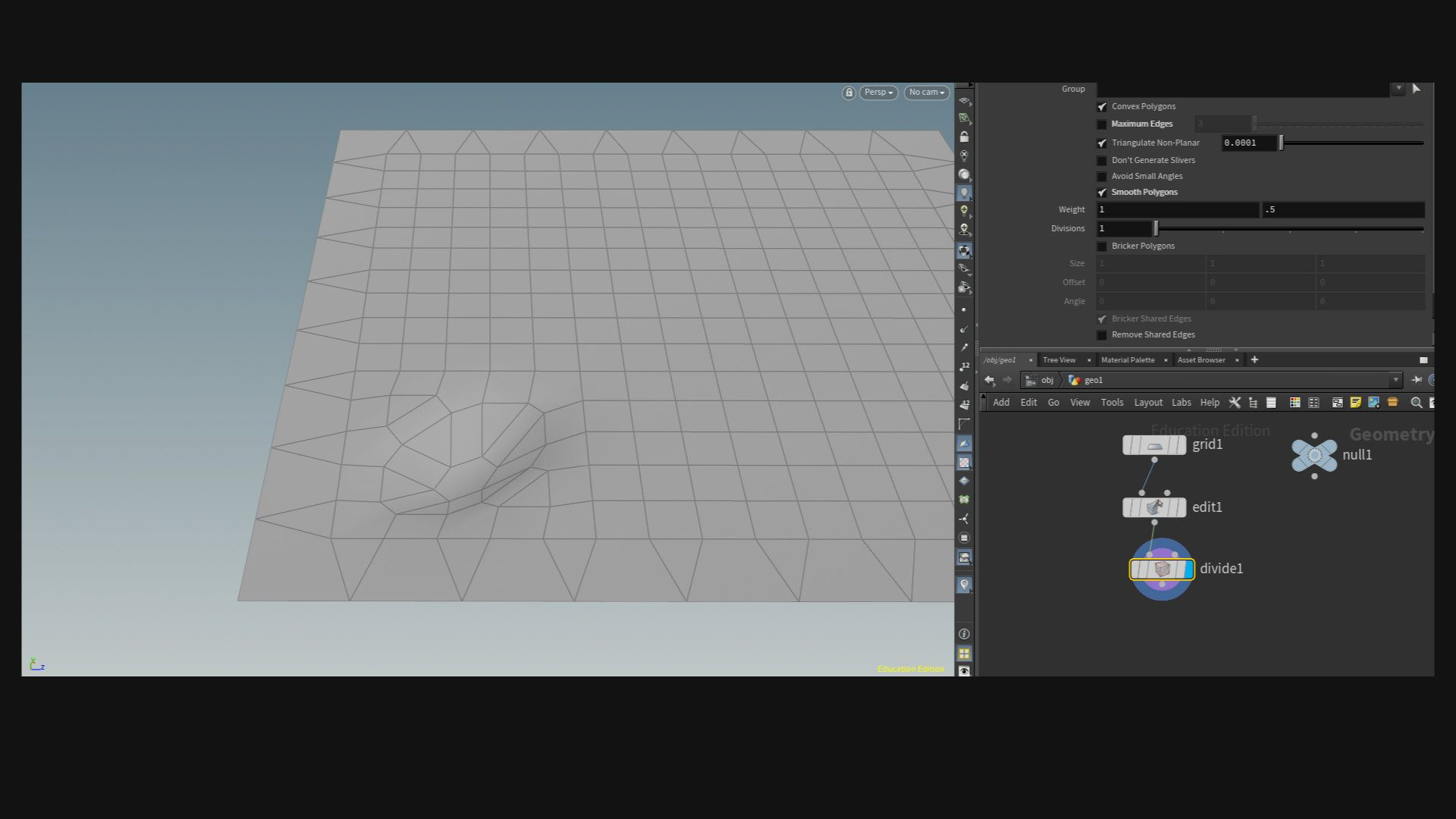-  Keep in mind that it will allow for N-Gons even if the "Maximum Edges" is turned off.  This can create weird looking topology that may be useful for things like chain-link fences or geometric patters.  As an example, if we triangulate the mesh, and then do a smooth operation, we'll get this: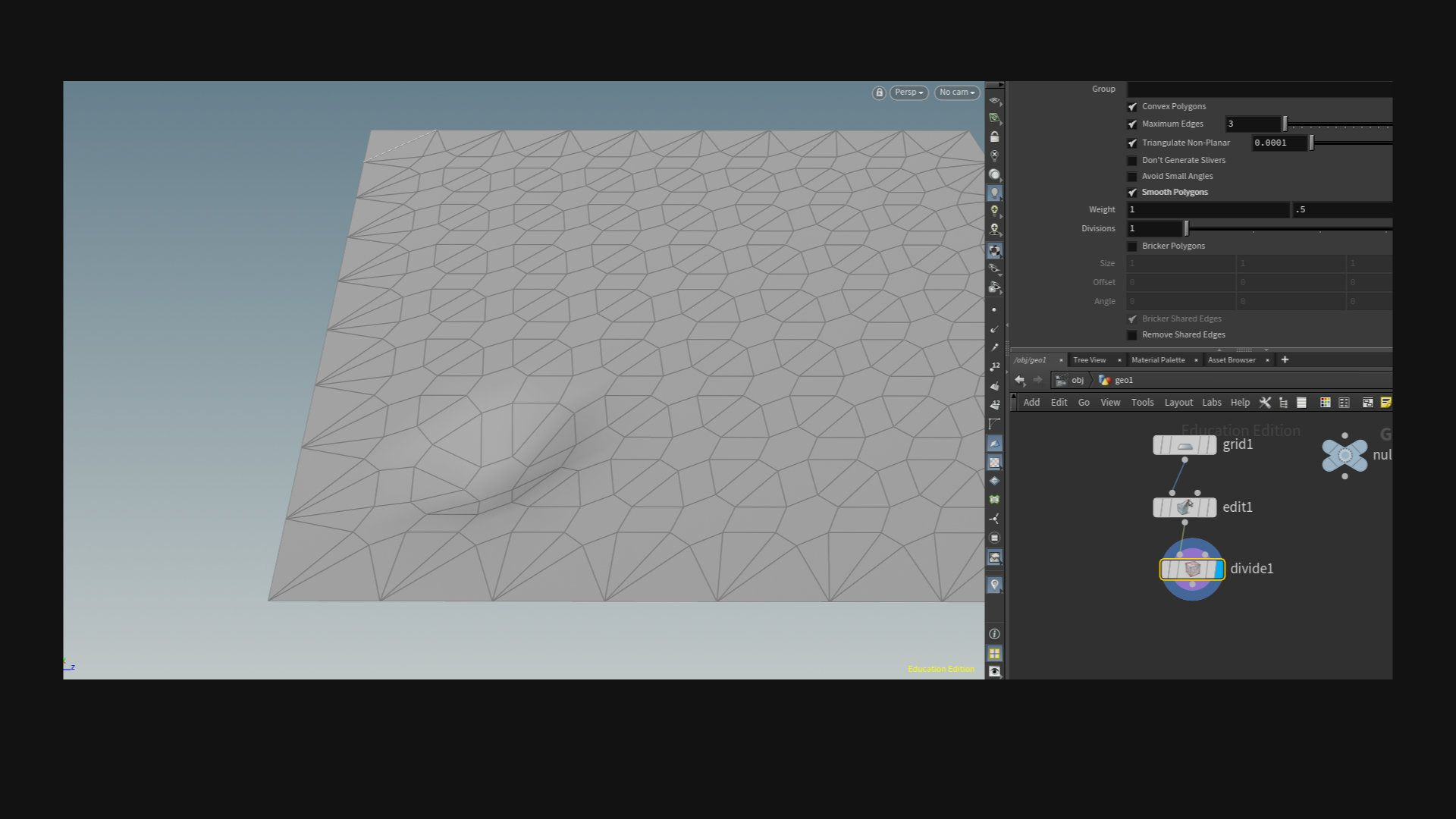-  Then, if we turn up the "weight" parameter, it will effectively tighten the topology: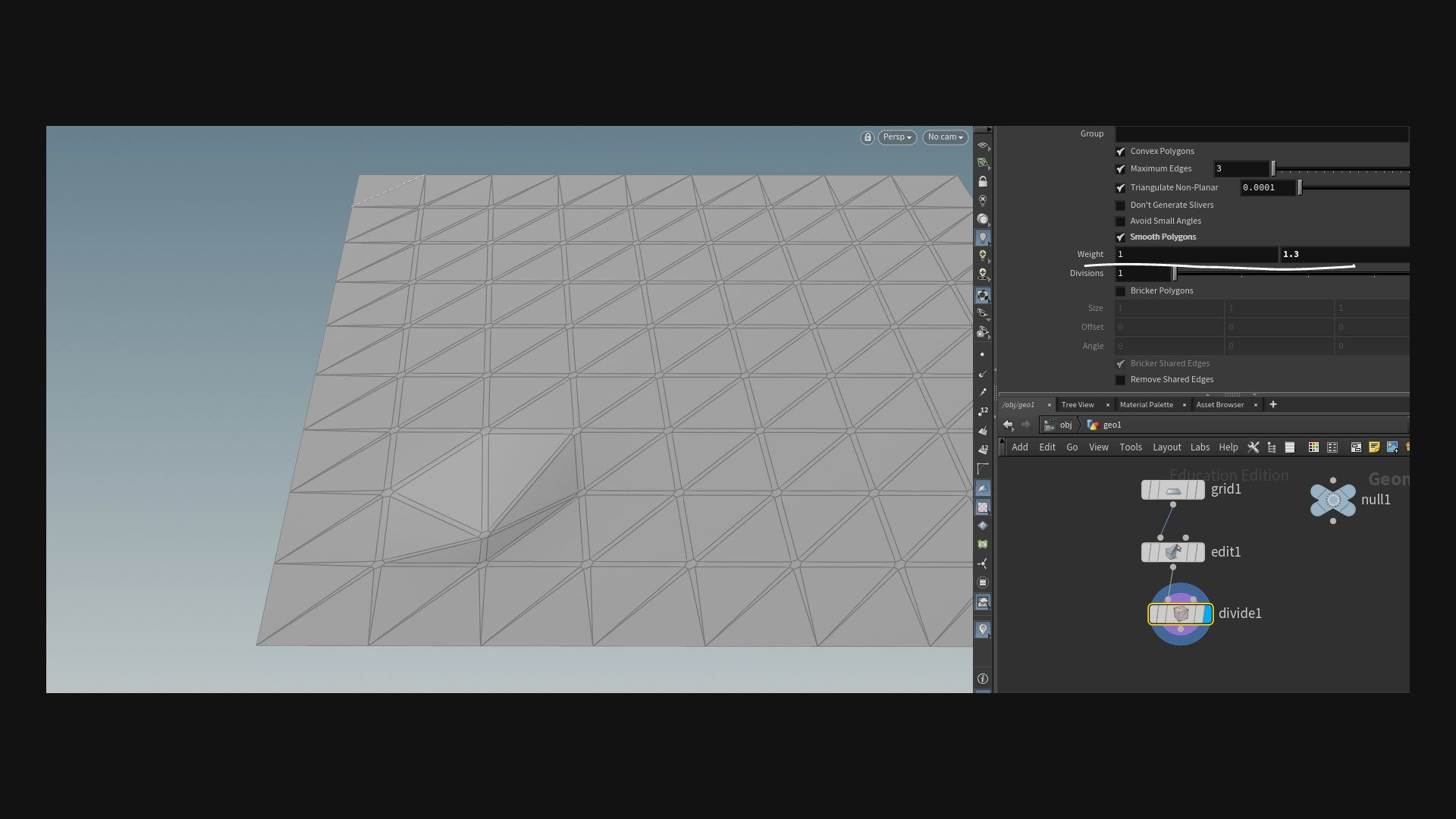Bricker Polygons:

-  This will create an evenly-spaced quad mesh over your existing topology.  In practice, this can be very useful for creating evenly-spaced topology in RBD simulations.  It's also useful for painting point attributes because it provides evenly-spaced points.  Keep in mind that this will generate N-Gons.  So, it's usually best to do a "Bricker" divide node followed by another divide node that triangulates the N-gons.  The size, offset, and angle will simply change the shape of that evenly-spaced quad topology.

Bricker Shared Edges:

-  According to the docs, "Controls if brickering will also divide the edges of polygons outside of the specified group, provided those edges were brickered inside the group. This prevents the brickering from creating gaps by inserting T-junctions in the topology."  In practice, it's fine to leave this on by default.

Remove Shared Edges:

-  This parameter is interesting because it will, by default remove most (or all of) your mesh.  But, if there are any polygons that have border edges (edges that are not shared by other polygons) then it will create a polygon based on those border edges.  In practice, this may be useful for identifying meshes that contain holes in it.  Or, if you'd like to create geometry based on those holes, this can do this as well.

Compute Dual:

-  According to the docs, "Convert the polyhedron into its point/face dual."  This unfortunately, is not very helpful for the average reader, so I will try to explain.  The "duality" of a polyhedron is described here: https://math.fandom.com/wiki/Dual_polyhedron

That's basically a bunch of math mumbo-jumbo for calculating the positions which correspond with the middle of the polygons, and it gives you a hexagon-shaped pattern that looks like this: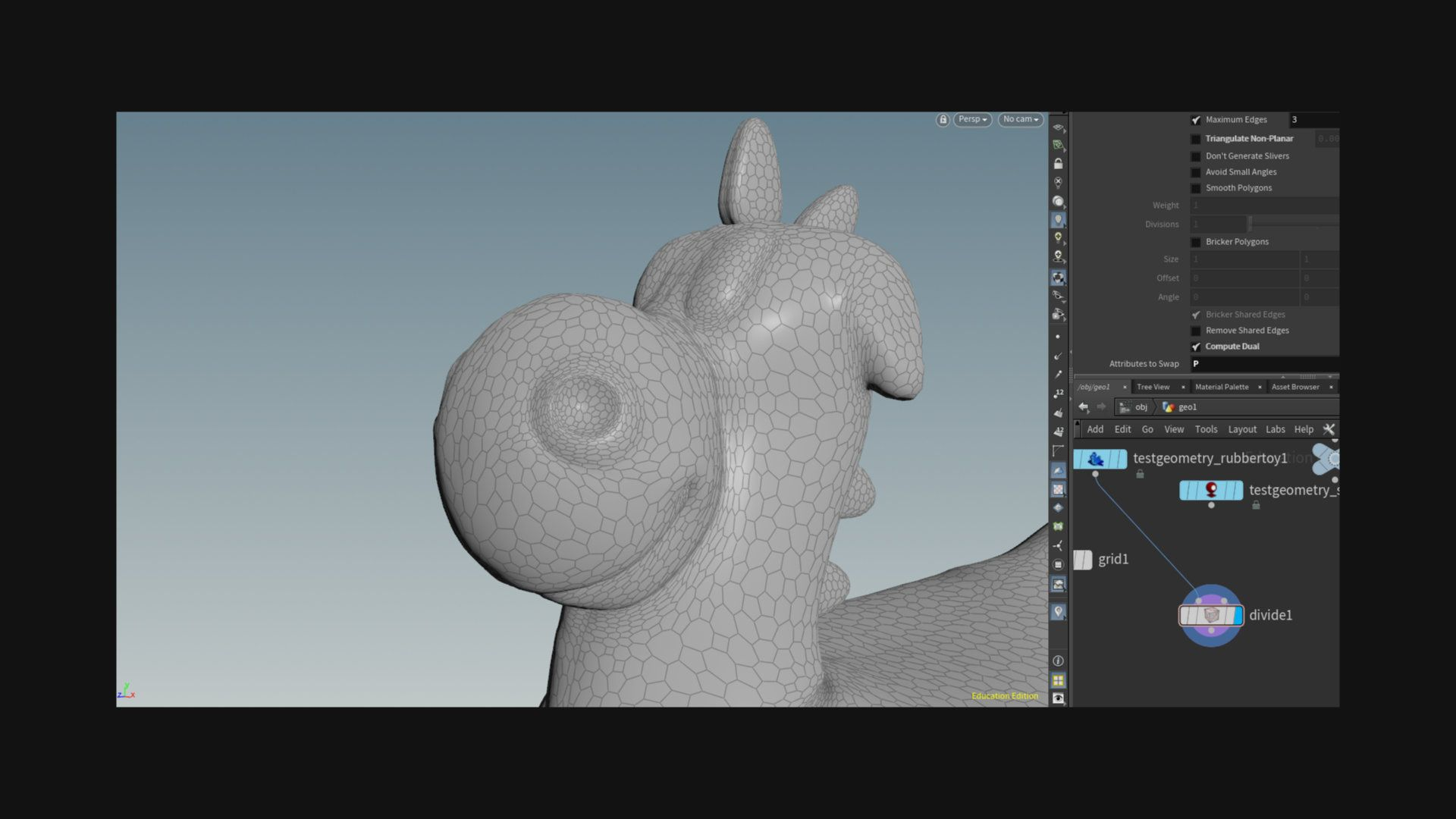-  For all intents and purposes, this is all you need to know about it unless you're trying to take this idea further.  And, if you do want to take it further, then also check out this blog post which describes it in more detail:  https://medium.com/@jakerice_7202/computing-the-dual-ff2a93077e2e

Attributes to Swap:

-  This parameter is responsible for transferring attributes from the old topology to the new hexagonal topology.  In general, leave this at its defaults

Drag to resize